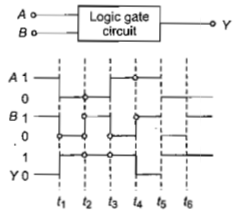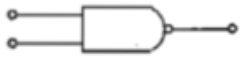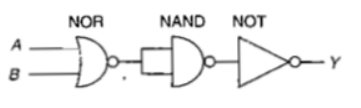The following figure shows a logic gate circuit with two inputs A and B and the output Y. The voltage waveforms of A, B and Y are as givenThe logic gate is

(a) $\mathrm{NOR}$ gate                                                 (b) $\mathrm{OR}$ gate

(c)  gate                                                 (d) gate

Concept Questions :-

Logic gates
High Yielding Test Series + Question Bank - NEET 2020

Difficulty Level:

For transistor action

(1) Base, emitter and collector regions should have similar size and doping concentrations.

(2) The base regions must be very thin and lightly doped.

(3) The emitter-base junction is forward biased and the base-collector junction is reverse biased.

(4) Both the emitter-base junction as well as the base-collector junction are forward biased.

Which of the following pairs of statements is correct?

(a)   (4) and (1)                                       (b)   (1) and (2)

(c)   (2) and (3)                                       (d)    (3) and (4)

Concept Questions :-

Transistor
High Yielding Test Series + Question Bank - NEET 2020

Difficulty Level:

A p-n photodiode is fabricated from a semiconductor with a band gap of  It can detect a signal of wavelength

(a)                                     (b)

(c)                                   (d)

Concept Questions :-

Applications of PN junction
High Yielding Test Series + Question Bank - NEET 2020

Difficulty Level:

The symbolic representation of four logic gates(i)                                                                              (ii)(iii)                                                                              (iv)

The logic symbols for $\mathrm{OR},$ $\mathrm{NOT}$ and $\mathrm{NAND}$ gates are respectively

(a)    (iii), (iv), (ii)                                            (b)   (iv), (i), (iii)

(c)    (iv), (ii), (i)                                              (d)   (i), (iii), (iv)

Concept Questions :-

Logic gates

Difficulty Level:

A transistor is operated in common-emitter configuration at ${V}_{c}=2volt$ such that a change in the base current from 100 $\mu A$ to 200 $\mu A$ produces a change in the collector current from 5 mA to 10 mA. The current gain is

1. 75                                2. 100

3. 150                              4. 50

Concept Questions :-

Transistor
High Yielding Test Series + Question Bank - NEET 2020

Difficulty Level:

Sodium has body centred packing.Distance between two nearest atom is $3.7\stackrel{0}{A}$. The lattice parameter is

(a) 6.8 $\stackrel{0}{A}$                                     (b) 4.3 $\stackrel{0}{A}$

(c) 3.0 $\stackrel{0}{A}$                                     (d) 8.6 $\stackrel{0}{A}$

Concept Questions :-

Types of semiconductors
High Yielding Test Series + Question Bank - NEET 2020

Difficulty Level:

If the lattice parameter for a crystalline structure is  then the atomic radius in fcc crystal is

(a)                                   (b)

(c)                                 (d)

Concept Questions :-

Types of semiconductors
High Yielding Test Series + Question Bank - NEET 2020

Difficulty Level:

The voltage gain of an amplifier with 9% negative feedback is 10. The voltage gain without feedback will be

(a) 90                            (b) 10

(c) 1.25                         (d) 100

Concept Questions :-

Applications of Transistor
High Yielding Test Series + Question Bank - NEET 2020

Difficulty Level:

A p-n photodiode is made of a material with a band gap of 2.0 eV. The minimum frequency of the radiation that can be absorbed by the material is nearly

1.                               2.

3.                                 4.

Concept Questions :-

Applications of PN junction
High Yielding Test Series + Question Bank - NEET 2020

Difficulty Level:

The circuit is equivalent to1. AND gate                              2. NAND gate

3. NOR gate                              4. OR gate

Concept Questions :-

Logic gates